Ex 1.3

Chapter 1 Class 7 Integers
Serial order wise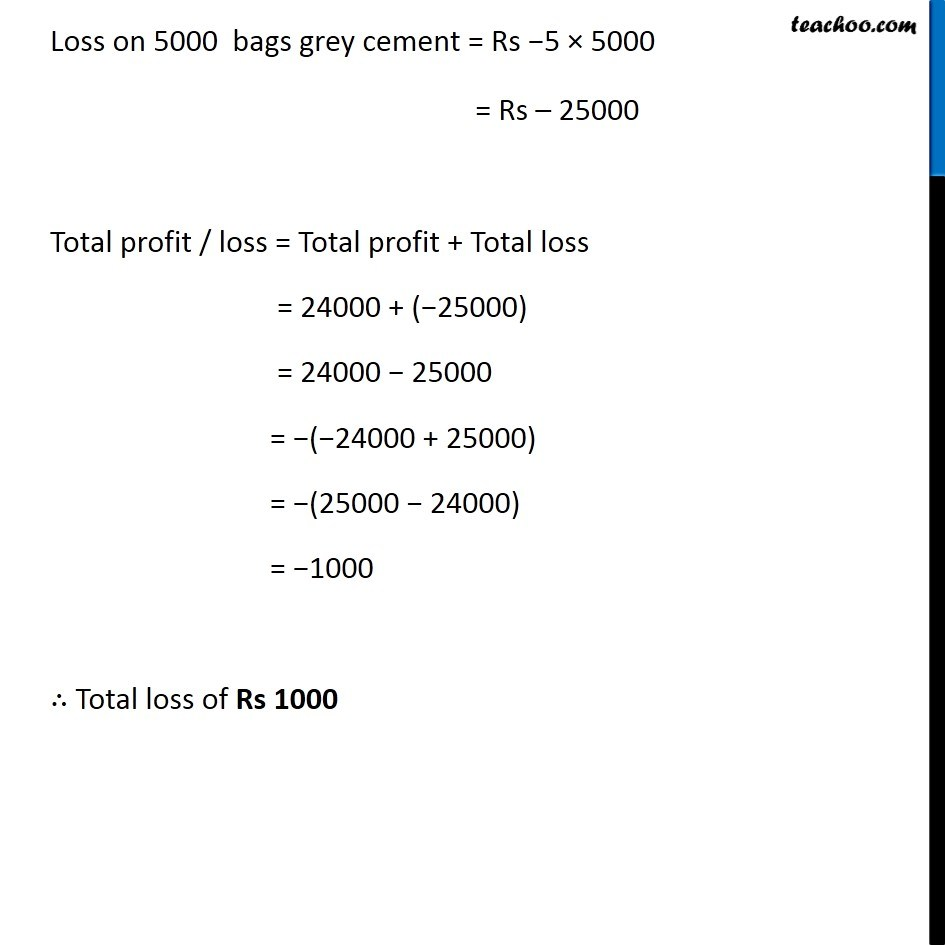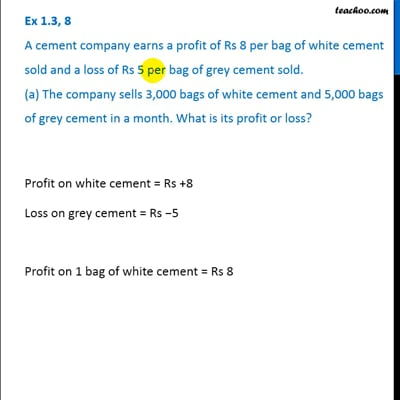This video is only available for Teachoo black users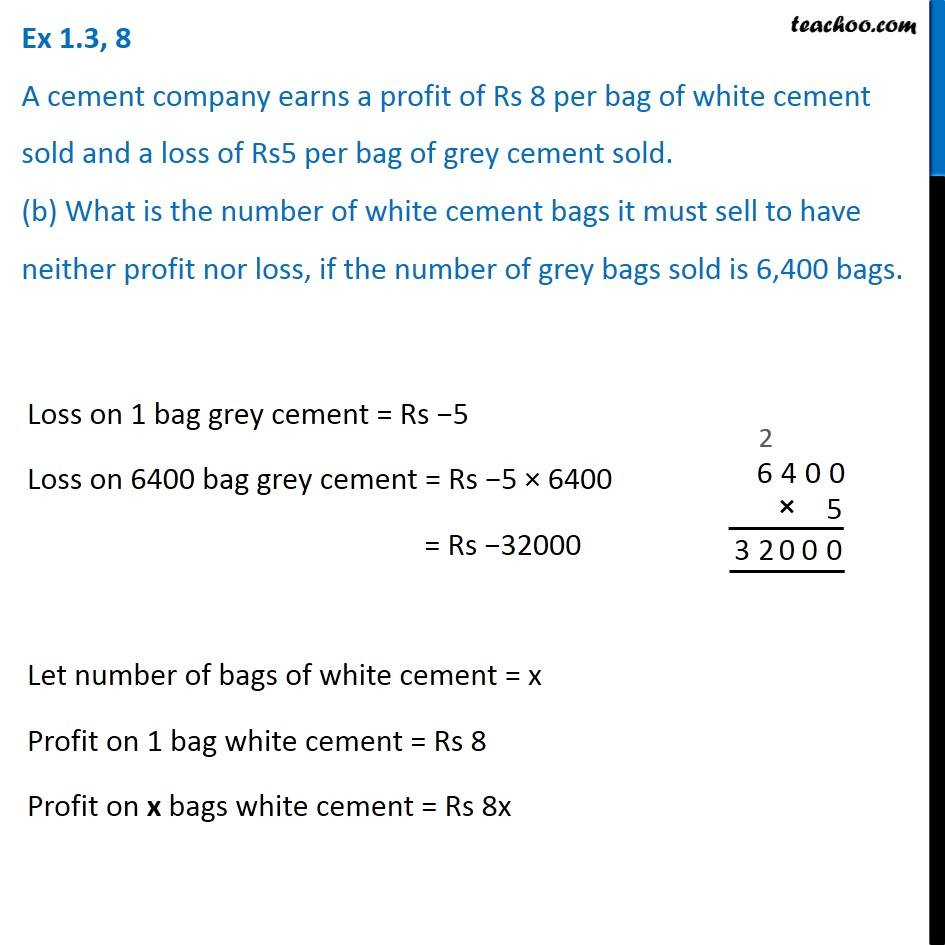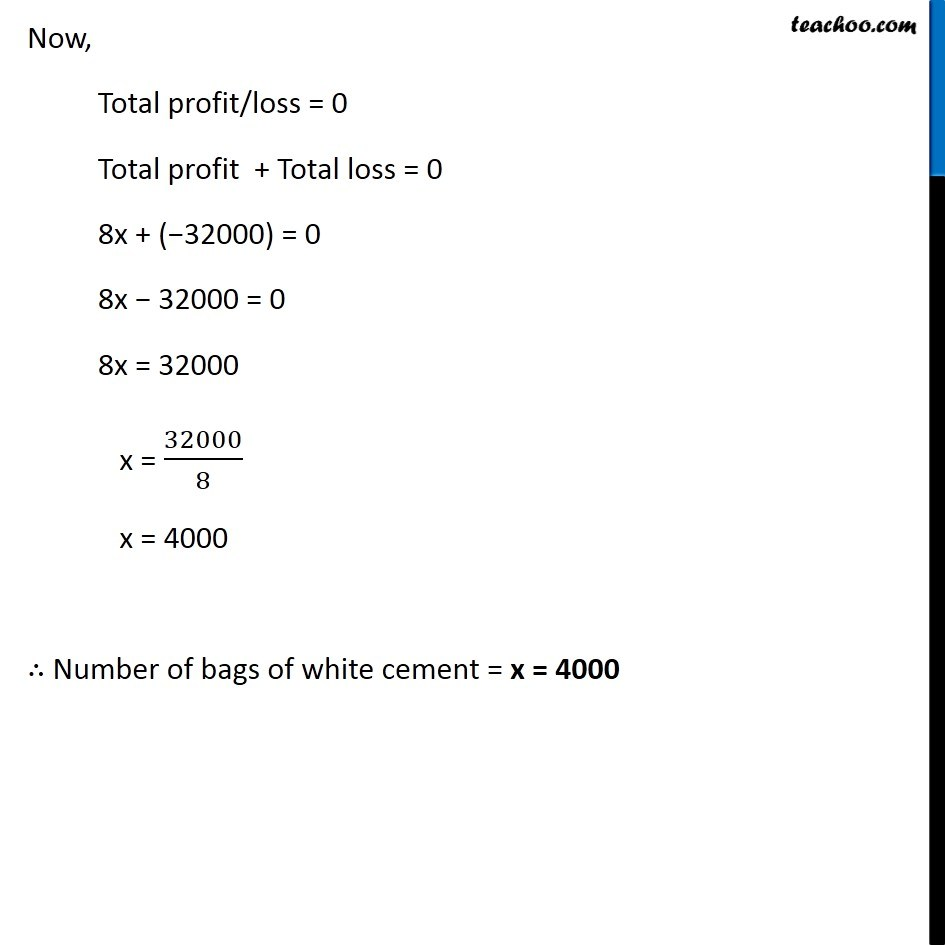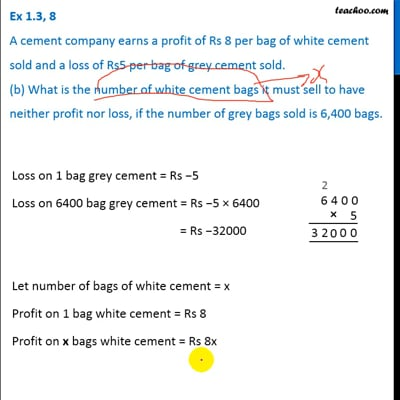This video is only available for Teachoo black users

### Transcript

Ex 1.3, 8 A cement company earns a profit of Rs 8 per bag of white cement sold and a loss of Rs 5 per bag of grey cement sold. (a) The company sells 3,000 bags of white cement and 5,000 bags of grey cement in a month. What is its profit or loss? Profit on white cement = Rs +8 Loss on grey cement = Rs −5 Profit on 1 bag of white cement = Rs 8 Profit on 3000 bags of white cement = Rs 8 × 3000 = Rs 24000 Loss on 1 bag grey cement = Rs −5 Loss on 5000 bags grey cement = Rs −5 × 5000 = Rs – 25000 Total profit / loss = Total profit + Total loss = 24000 + (−25000) = 24000 − 25000 = −(−24000 + 25000) = −(25000 − 24000) = −1000 ∴ Total loss of Rs 1000 Ex 1.3, 8 A cement company earns a profit of Rs 8 per bag of white cement sold and a loss of Rs5 per bag of grey cement sold. (b) What is the number of white cement bags it must sell to have neither profit nor loss, if the number of grey bags sold is 6,400 bags.Loss on 1 bag grey cement = Rs −5 Loss on 6400 bag grey cement = Rs −5 × 6400 = Rs −32000 Let number of bags of white cement = x Profit on 1 bag white cement = Rs 8 Profit on x bags white cement = Rs 8x Now, Total profit/loss = 0 Total profit + Total loss = 0 8x + (−32000) = 0 8x − 32000 = 0 8x = 32000 x = 32000/8 x = 4000 ∴ Number of bags of white cement = x = 4000